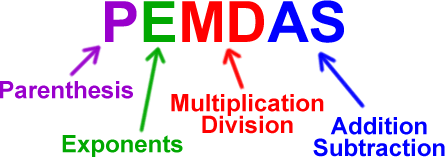Order of Operation
3 years ago
ldelfeld
Save
Edit
Host a game
Live GameLive
Homework
Solo Practice
Practice18 QuestionsShow answers
• Question 1
120 seconds
Q. (2 + 2) - 1
4
2
3
• Question 2
120 seconds
Q. 14 + (22 - 2) =
34
37
40
• Question 3
180 seconds
Q. 20 x 2 - (10 - 4)
22
30
34
• Question 4
120 seconds
Q. (23 + 24) -10 =
37
27
47
• Question 5
120 seconds
Q. (2 x 5) + 18
48
20
28
• Question 6
30 seconds
Q. Which operation is performed FIRST?
(13 - 1) + 4
subtraction
multiplication
• Question 7
30 seconds
Q. Which operation is performed SECOND?
(13 - 1) + 4
subtraction -
multiplication X
• Question 8
30 seconds
Q. Which operation is performed FIRST?
25 x (3-1)
multiplication
subtraction
division
• Question 9
30 seconds
Q. Which operation is performed SECOND?
44 ÷ 2 X (3 -1)
muliplication X
division ÷
subtraction -
• Question 10
30 seconds
Q. Which operation is performed FIRST?
44 ÷ 2 X (3 -1)
muliplication X
division ÷
subtraction -
• Question 11
30 seconds
Q. Which operation is performed SECOND?
(32 ÷ 8) + 27 - 11
division ÷
subtraction -
• Question 12
120 seconds
Q. Solve:
(32 ÷ 8) + 27 - 11
10
20
30
• Question 13
30 seconds
Q. Which operation is performed SECOND?
(40 x 4) - 33
multiplication X
subtraction
division ÷
• Question 14
120 seconds
Q. Solve:
(40 x 4) - 33
100
125
127
• Question 15
30 seconds
Q. What does the E in PEMDAS stand for?
Exceptional
Egg
Exponent
• Question 16
30 seconds
Q. Which operation is performed FIRST:
38 + (9 x 6) ÷ 3
division ÷
multiplication X
• Question 17
30 seconds
Q. Which operation is performed SECOND :
38 + (9 x 6) ÷ 3
multiplication